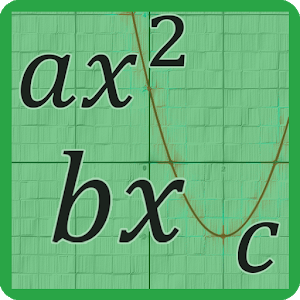Ελληνικά

Κάθε μέρα προσφέρουμε ΔΩΡΕΑΝ εφαρμογές και παιχνίδια με άδεια χρήσης για Android που θα έπρεπε να αγοράσετε σε άλλη περίπτωση.\$1.48
ΕΛΗΞΕ

# Android Giveaway of the Day - Quadratic Equation Solver PRO

This is a smart application which solves quadratic equations or formulas and gives you the step-by-step solution.
\$1.48 ΕΛΗΞΕ
Αξιολόγηση Χρηστών: 0 (0%) 0 (0%)

This giveaway offer has been expired. Quadratic Equation Solver PRO is now available on the regular basis.

This is a smart application which solves quadratic equations or formulas and gives you the step-by-step solution. Unlike most of the other apps, this app is featured with both "Quadratic Formula" and "Completing the square" methods.

★ Capable of generating graphs for a given equation.

★ Both "Quadratic Formula" and "Completing the square" methods available.

★ Ability to save step-by-step solution as an image.

★ User friendly interface with Material design.

★ Decimal and fractional number inputs.

★ Decimal and fractional number output.

★ Handles imaginary numbers.

★ Each variable input is a simple calculator supporting the following operators ( *, /, +, -).

★ Lightweight.

NOTE : Quadratic equations are of the form ax2+bx+c=0 where a, b and c are real numbers and "a" should not be equal to zero. Quadratic equations have two solutions. It is possible that one solution may repeat. You can calculate quadratic equations by Completing the square and by Quadratic Formula.

Solving by Competing the Square

• Keep all terms containing x on one side. Move the constant to the right.

• Get ready to create a perfect square on the left. Balance the equation.

• Take half of the x-term coefficient and square it. Add this value to both sides.

• Simplify and write the perfect square on the left.

• Take the square root of both sides. Be sure to allow for both plus and minus.

• Solve for x.

The solutions of some quadratic equations are not rational, and cannot be factored. For such equations, the most common method of solution is the quadratic formula. The quadratic formula can be used to solve ANY quadratic equation.

K. Dev

Education

1.4.2

2.1M

Everyone

4.1 and up

## Σχόλια σχετικά με το Quadratic Equation Solver PRO

Thank you for voting!